$$\def\loading{......LOADING......Please Wait} \def\RR{\bf R} \def\real{\mathbb{R}} \def\bold#1{\bf #1} \def\d{\mbox{Cord}} \def\hd{\widehat \mbox{Cord}} \DeclareMathOperator{\cov}{cov} \DeclareMathOperator{\var}{var} \DeclareMathOperator{\cor}{cor} \newcommand{\ac}{\left\{#1\right\}} \DeclareMathOperator{\Ex}{\mathbb{E}} \DeclareMathOperator{\diag}{diag} \newcommand{\bm}{\boldsymbol{#1}} \def\wait{......LOADING......Please Wait}$$

## Some Machine Learning Methods for NeuroImaging Analysis

### Xi (Rossi) LUO

The University of Texas
Health Science Center
School of Public Health
Dept of Biostatistics
and Data Science

Houston, Texas
September 17, 2019

Funding: NIH R01EB022911, P01AA019072, P20GM103645, P30AI042853; NSF/DMS (BD2K) 1557467

# Slides viewable on web:

## Overall Theme

• Machine learning: develop new models
• Optimization: fast and large-scale computation
• Theory: some theoretic properties
• Neuroimaging: big and complex data

# Granger Mediation Analysis

## Co-AuthorYi Zhao
Tenure-track Assistant Prof at Indiana Univ

## fMRI Experiments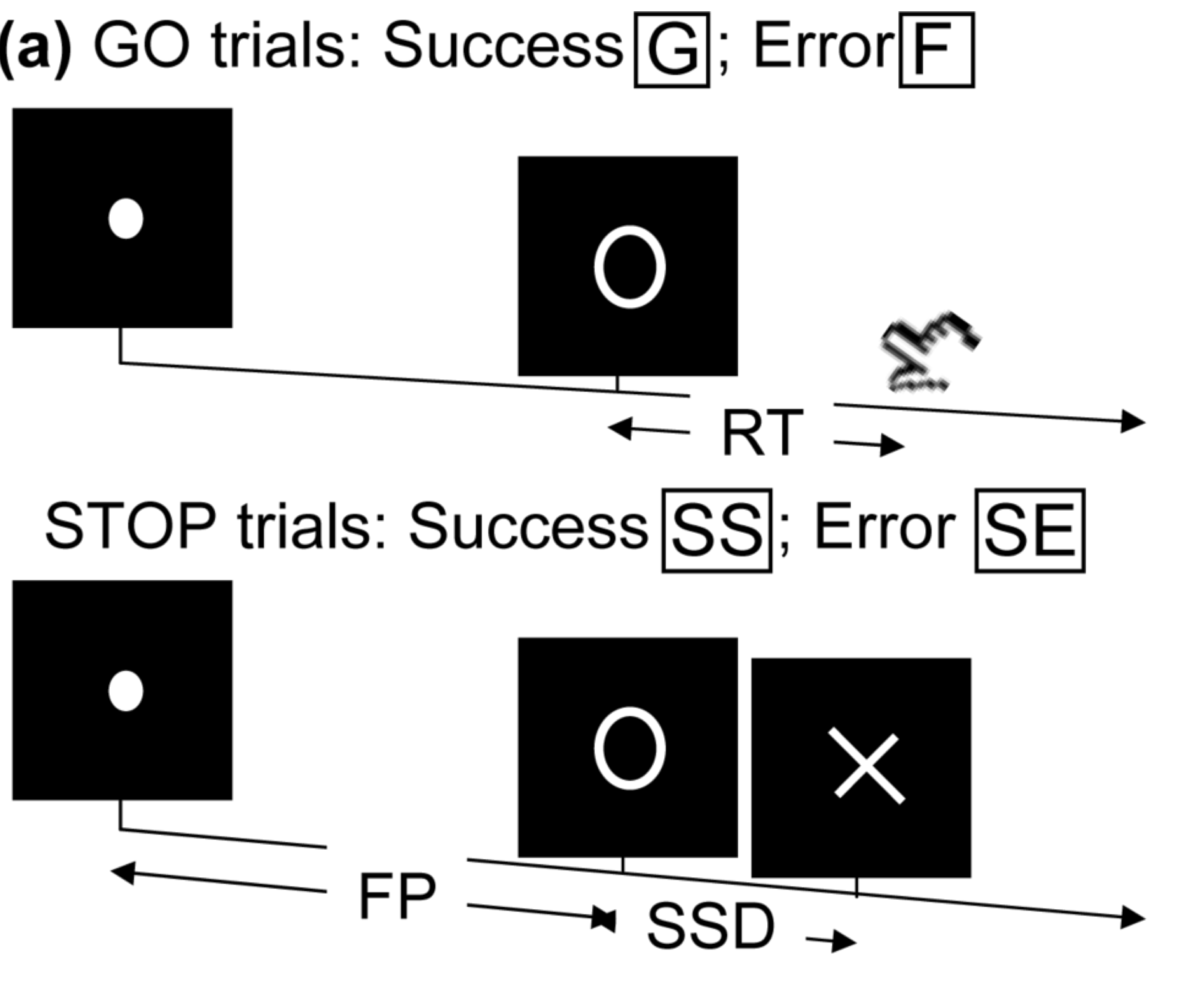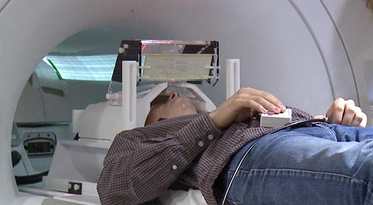• Task fMRI: performs tasks under brain scanning
• Randomized stop/go task:
• press button if "go";
• withhold pressing if "stop"
• Not resting-state: "do nothing" during scanning

Goal: infer brain activation and connectivity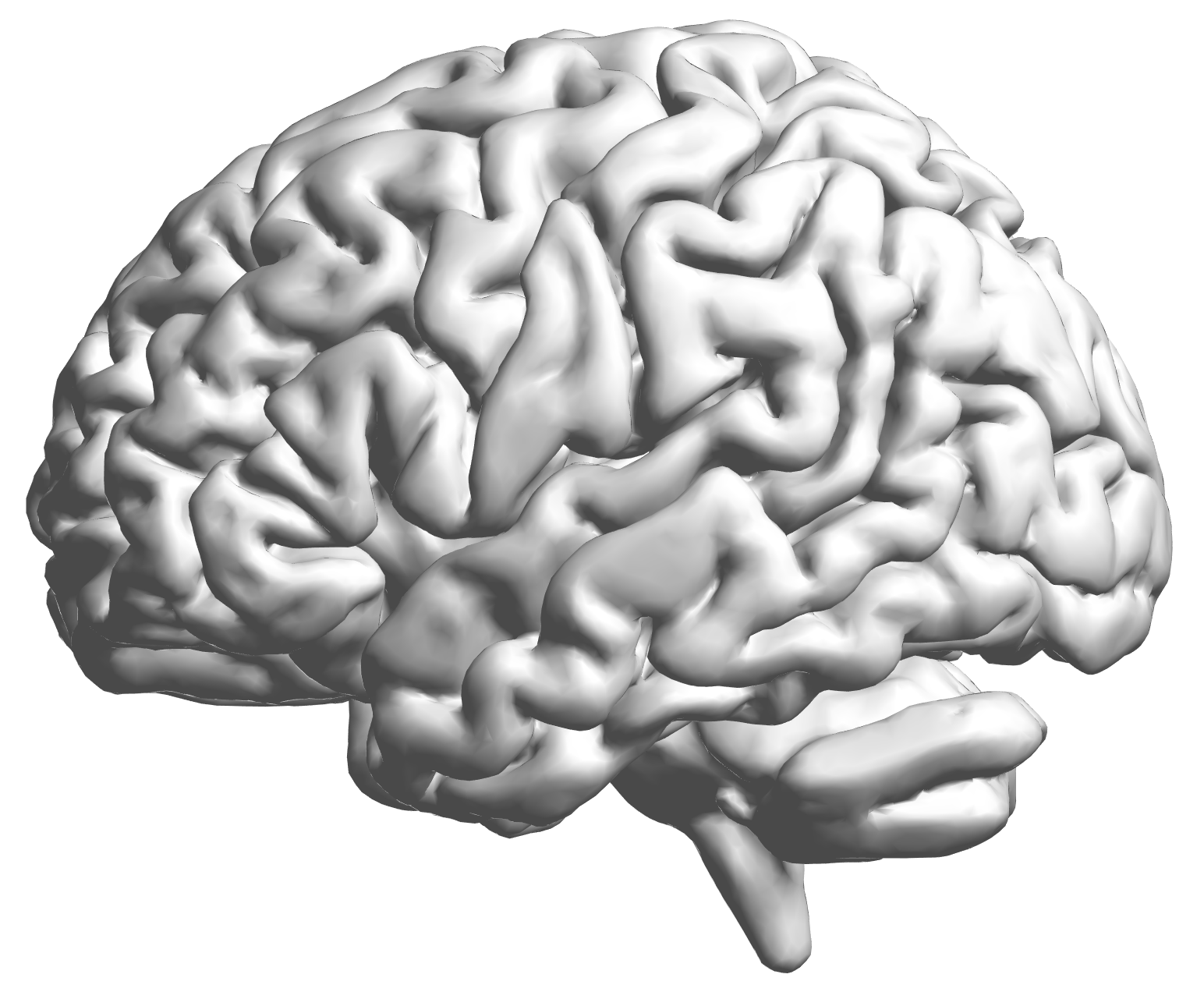fMRI data: blood-oxygen-level dependent (BOLD) signals from each cube/voxel (~millimeters), $10^5$ ~ $10^6$ voxels in total.

## Multilevel fMRI Studies

Sub 1, Sess 1Time 12~200

Sub i, Sess jSub ~100, Sess ~4Large, multilevel (subject, session, voxel) data
e.g. $1000 \times 4 \times 300 \times 10^6 \approx 1$ trillion data points

## Raw Data: Motor Region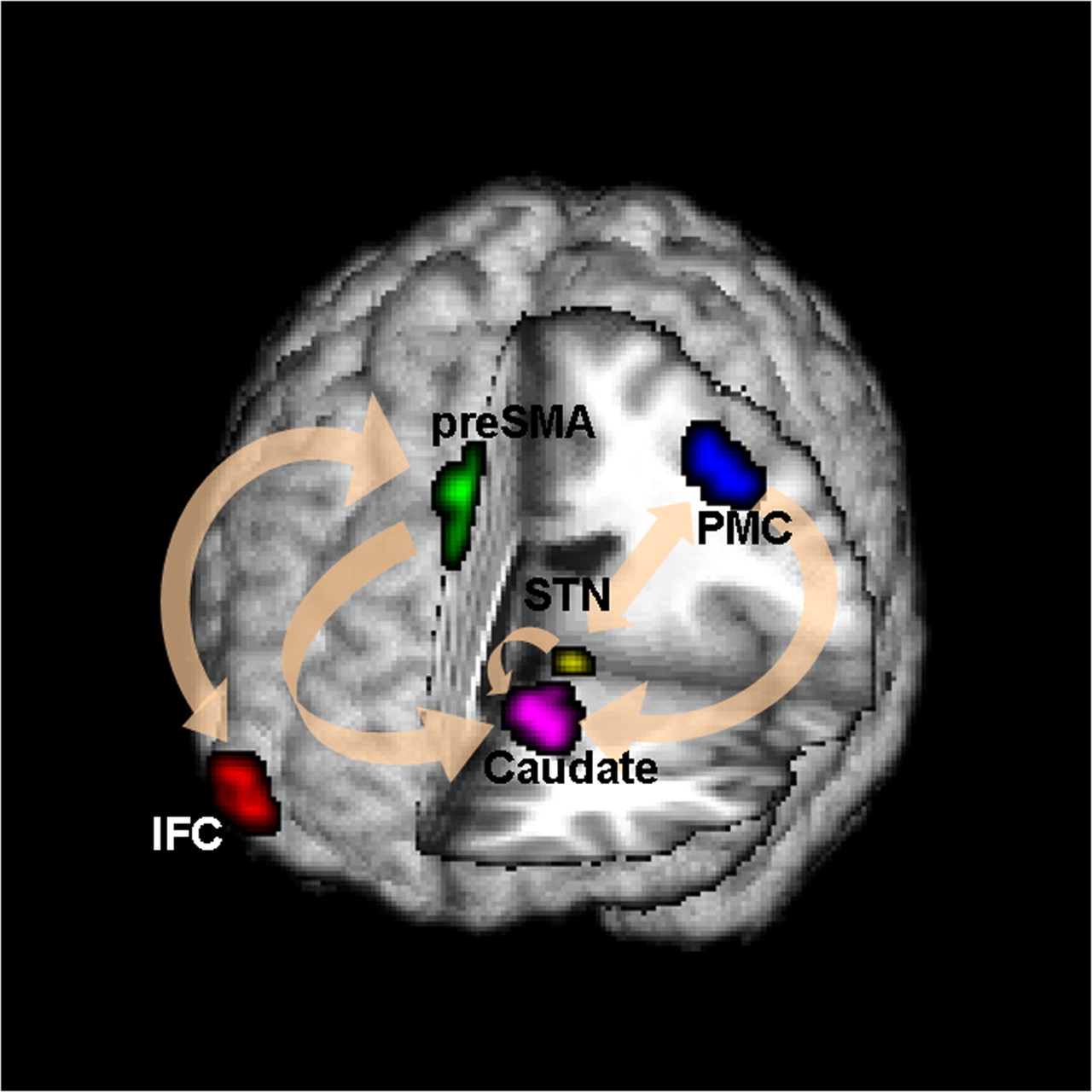Time (seconds)

Black: fMRI BOLD activity
Blue: stop onset times; Red: go onset times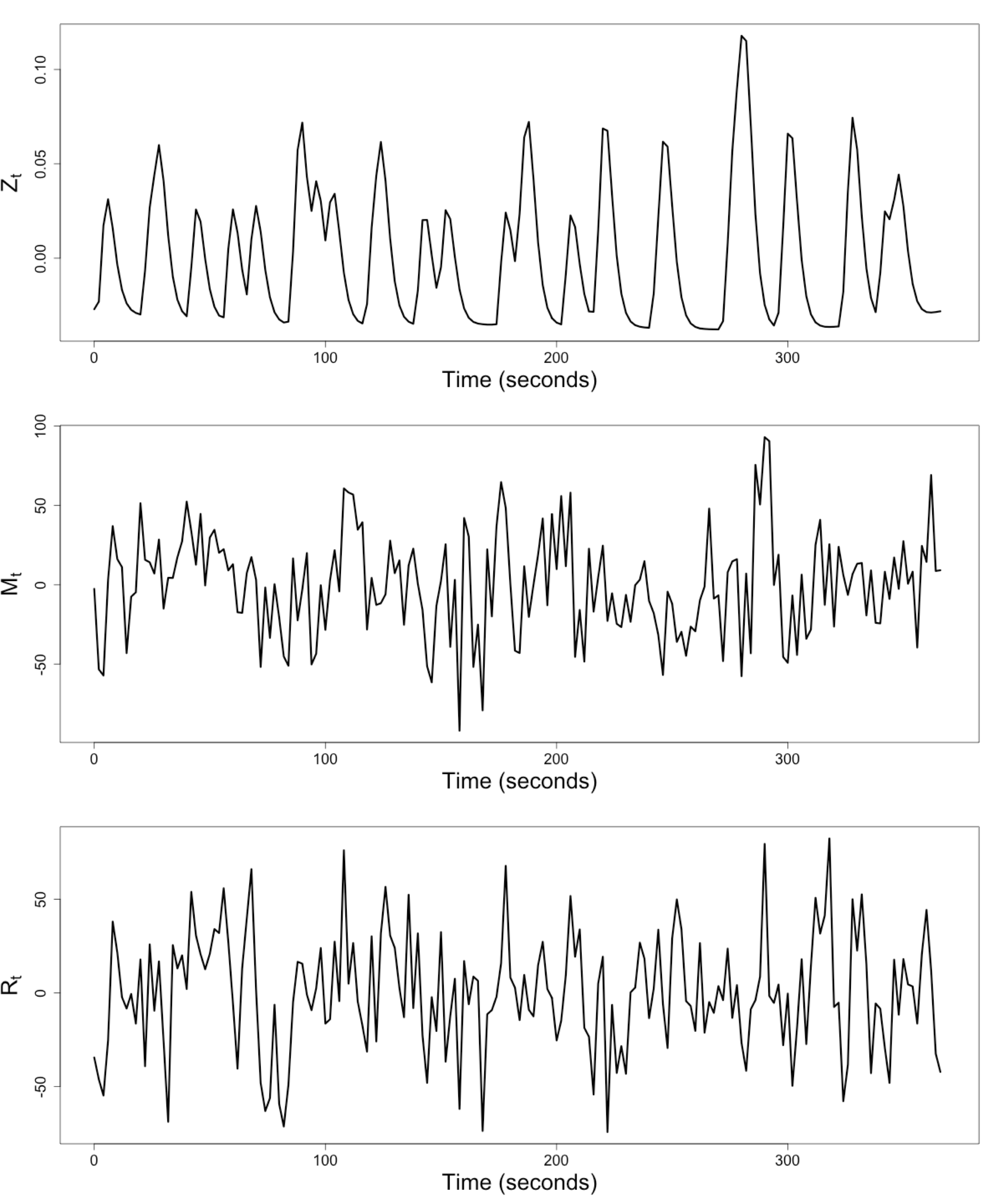$Z_t$: Stimulus onsets convoluted with Canonical HRF

$M_t$, $R_t$: fMRI time series from two brain regions

## Review: Granger Causality/VAR

• Given two (or more) time series $x_t$ and $y_t$ \begin{align*} x_t &= \sum_{j=1}^p \psi_{1j} x_{t-j} + \sum_{j=1}^p \phi_{1j} y_{t-j} + \epsilon_{1t} \\ y_t &= \sum_{j=1}^p \psi_{2j} y_{t-j} + \sum_{j=1}^p \phi_{2j} x_{t-j} + \epsilon_{2t} \end{align*}
• Also called vector autoregressive models
• $y$ Granger causes $x$ if $\phi_{1j} \ne 0$ Granger, 69
• Models pair-wise connections not pathways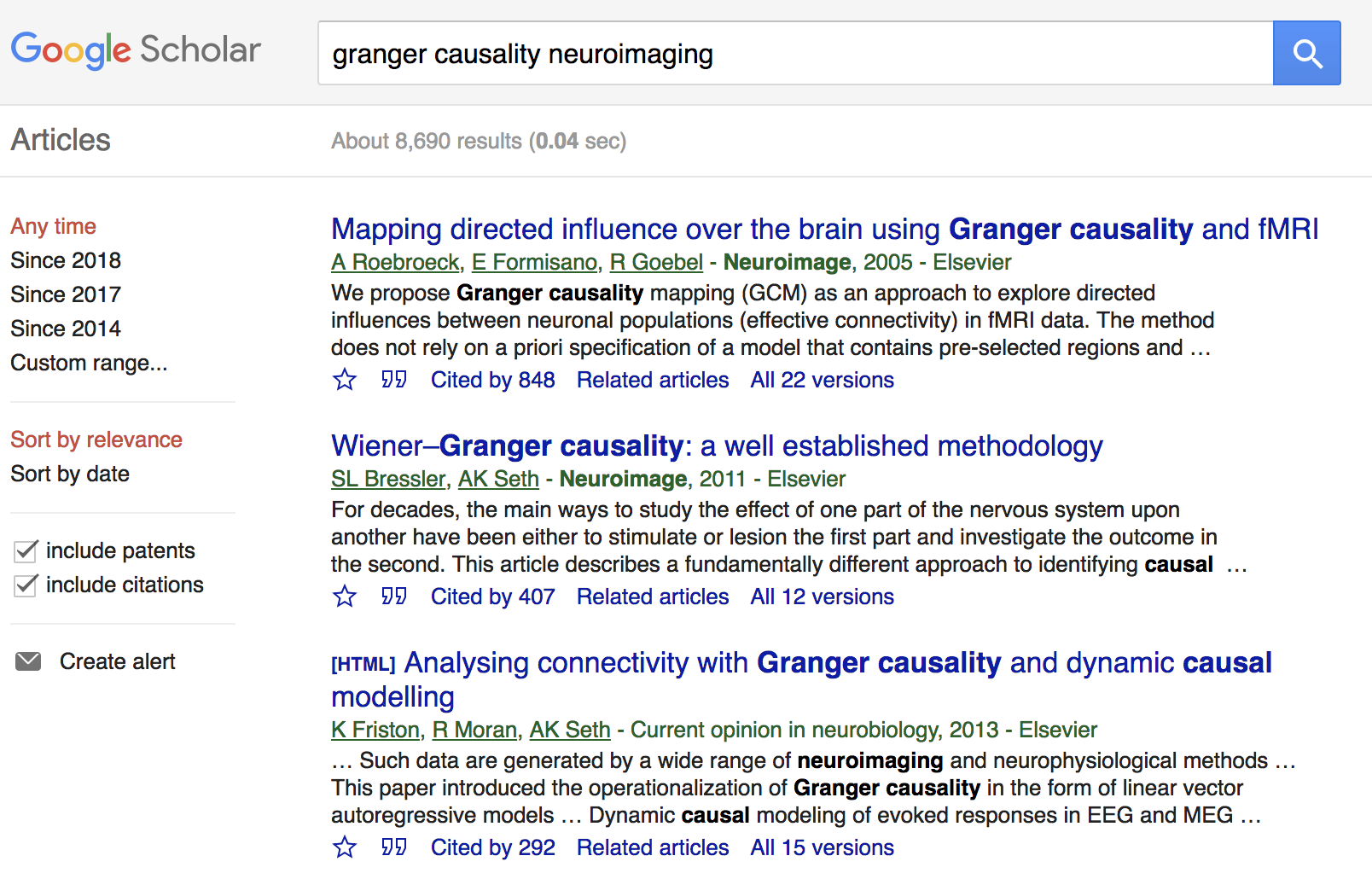## Granger Causality/VAR

• Granger Causality (VAR) popular for fMRI
• Over 10,000 google scholar results on "granger causality neuroimaging", as of May 29, 2019
• Models multiple stationary time series
• AR($p$) (small $p$) fits fMRI well Lingdquist, 08
• Not for non-stationary/task fMRI
• Cannot model stimulus effects

## Conceptual Brain Model with StimulusGoal: quantify effects stimulipreSMAPMC regions Duann, Ide, Luo, Li (2009). J of Neurosci

## Model: Mediation Analysis and SEM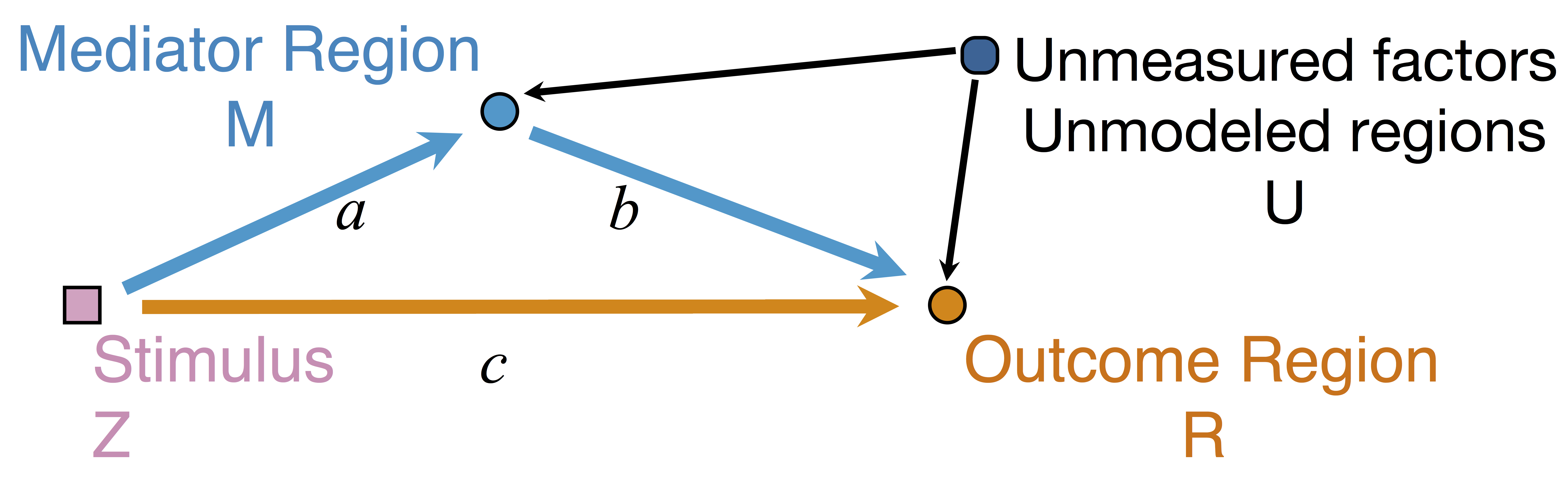\begin{align*}M &= Z a + \overbrace{U + \epsilon_1}^{E_1}\\ R &= Z c + M b + \underbrace{U g + \epsilon_2}_{E_2}, \quad \epsilon_1 \bot \epsilon_2\end{align*}
• Indirect effect: $a \times b$; Direct effect: $c$
• Correlated errors: $\delta = \cor(E_1, E_2) \ne 0$ if $U\ne 0$

## Mediation Analysis in fMRI

• Mediation analysis (usually assuming $U=0$)Baron&anp;Kenny, 86; Sobel, 82; Holland 88; Preacher&Hayes 08; Imai et al, 10; VanderWeele, 15;...
• Parametric Wager et al, 09 and functional Lindquist, 12 mediation, under (approx.) independent errors
• Stimulus $\rightarrow$ brain $\rightarrow$ user reported ratings, one brain mediator
• Assuming $U=0$ between ratings and brain
• Multiple mediator and multiple pathways
• Dimension reduction by arXiv1511.09354Chen, Crainiceanu, Ogburn, Caffo, Wager, Lindquist, 15
• Pathway Lasso penalization Zhao, Luo, 16
• This talk: integrating Granger causality and mediation analysis

# Model & Method

## Our Mediation Model

\begin{align*}M_{t} = Z_{t} a + E_{1t},\quad R_t = Z_t c + M_t b + E_{2t}\end{align*}
• Temporal VAR errors \begin{align*} E_{1t}&=& \sum_{j=1}^{p}\left(\omega_{11_{j}}E_{1,t-j}+\omega_{21_{j}}E_{2,t-j}\right)+\epsilon_{1t} \\ E_{2t}&=&\sum_{j=1}^{p}\left(\omega_{12_{j}}E_{1,t-j}+\omega_{22_{j}}E_{2,t-j}\right)+\epsilon_{2t} \end{align*}
• Spatial errors: $\epsilon_{1t}, \epsilon_{2t}$ $$\begin{pmatrix} \epsilon_{1t} \\ \epsilon_{2t} \end{pmatrix}\sim\mathcal{N}\left(\boldsymbol{\mathrm{0}},\boldsymbol{\Sigma}\right), \quad \boldsymbol{\Sigma}=\begin{pmatrix} \sigma_{1}^{2} & \delta\sigma_{1}\sigma_{2} \\ \delta\sigma_{1}\sigma_{2} & \sigma_{2}^{2} \end{pmatrix}$$

## Equivalent Form

\begin{align*} \scriptsize M_{t}& \scriptsize =Z_{t}A+\sum_{j=1}^{p}\left(\phi_{1j}Z_{t-j}+\psi_{11_{j}}M_{t-j}+\psi_{21_{j}}R_{t-j}\right)+\epsilon_{1t} \\ \scriptsize R_{t}& \scriptsize =Z_{t}C+M_{t}B+\sum_{j=1}^{p}\left(\phi_{2j}Z_{t-j}+\psi_{12_{j}}M_{t-j}+\psi_{22_{j}}R_{t-j}\right)+\epsilon_{2t} \end{align*}
• Nonzero $\phi$'s and $\psi$'s denote the temporal influence from stimulus to mediator/outcome and etc
• $A$, $B$, $C$ are causal following a similar proof in Sobel, Lindquist, 04

## Causal Conditions

• The treatment randomization regime is the same across time and participants.
• Models are correctly specified, and no treatment-mediator interaction.
• At each time point $t$, the observed outcome is one realization of the potential outcome with observed treatment assignment $\mathbf{\bar S}_{t}$, where $\mathbf{ \bar S}_{t}=( \mathbf{S}_{1},\dots,\mathbf{S}_{t})$.
• The treatment assignment is random across time.
• The causal effects are time-invariant.
• The time-invariant covariance matrix is not affected by the treatment assignments.

## Estimation: Conditional Likelihood

• The full likelihood for our model is too complex
• Given the initial $p$ time points, the conditional likelihood is \begin{align*} & \tiny \ell\left(\boldsymbol{\Theta},\delta~|~\mathbf{Z},\mathcal{I}_{p}\right) = \sum_{t=p+1}^{T}\log f\left((M_{t},R_{t})~|~\mathbf{X}_{t}\right) \\ & \tiny = -\frac{T-p}{2}\log\sigma_{1}^{2}\sigma_{2}^{2}(1-\delta^{2})-\frac{1}{2\sigma_{1}^{2}}\|\mathbf{M}-\mathbf{X}\boldsymbol{\theta}_{1}\|_{2}^{2} \\ & \tiny -\frac{1}{2\sigma_{2}^{2}(1-\delta^{2})}\|(\mathbf{R}-\mathbf{M}B-\mathbf{X}\boldsymbol{\theta}_{2})-\kappa(\mathbf{M}-\mathbf{X}\boldsymbol{\theta}_{1})\|_{2}^{2} \end{align*}

## Multilevel Data: Two-level Likelihood

• Second level model, for each subject $i$ $$(A_i,B_i,C_i) = (A,B,C) + (\eta^A_i, \eta^B_i, \eta^C_i)$$ where errors $\eta$ are normally distributed
• The two level likelihood is conditional convex
• Two-stage fitting: plug-in estimates from the first level
• Block coordinate fitting: jointly optimize first level likelihood + second level likelihood
Theorem: Assume assumptions (A1)-(A6) are satisfied. Assume $\mathbb{E}(Z_{i_{t}}^{2})=q\lt \infty$, for $i=1,\dots,N$. Let $T=\min_{i}T_{i}$.
1. If $\boldsymbol{\Lambda}$ is known, then the two-stage estimator $\hat{\delta}$ maximizes the profile likelihood of model asymptotically, and $\hat{\delta}$ is $\sqrt{NT}$-consistent.
2. If $\boldsymbol{\Lambda}$ is unknown, then the profile likelihood of model has a unique maximizer $\hat{\delta}$ asymptotically, and $\hat{\delta}$ is $\sqrt{NT}$-consistent, provided that $1/\varpi=\bar{\kappa}^{2}/\varrho^{2}=\mathcal{O}_{p}(1/\sqrt{NT})$, $\kappa_{i}=\sigma_{i_{2}}/\sigma_{i_{1}}$, $\bar{\kappa}=(1/N)\sum\kappa_{i}$, and $\varrho^{2}=(1/N)\sum(\kappa_{i}-\bar{\kappa})^{2}$.
Using the two-stage estimator $\hat{\delta}$, the CMLE of our model is consistent, as well as the estimator for $\mathbf{b}=(A,B,C)$.

## Theory: Summary

• Under regularity conditions, $N$ subs, $T$ time points
• Our $\hat \delta$ is $\sqrt{NT}$-consistent
• This relaxes the unmeasured confounding assumption in mediation analysis
• Our $(\hat{A},\hat{B}, \hat{C})$ is also consistent

# Simulations & Real Data

## Comparison

• Our methods: GMA-h and GMA-ts
• Previous methods: BK Baron & Kenny, MACC Zhao and Luo, KKB Kenny et al
• Other methods do not model the temporal correlations or time series like ours

## SimulationsLow bias for $AB$Low bias for temporal cor

Gray dash lines are the truth

GMA performs the best, and recovers the temporal correlations

## Real Data Experiment

• Public data: OpenFMRI ds30
• Stop-go experiment: withhold (STOP) from pressing buttons
• Expect "STOP" stimuli to deactivate brain region M1
• Goal: quantify the role of region preSMA

## Result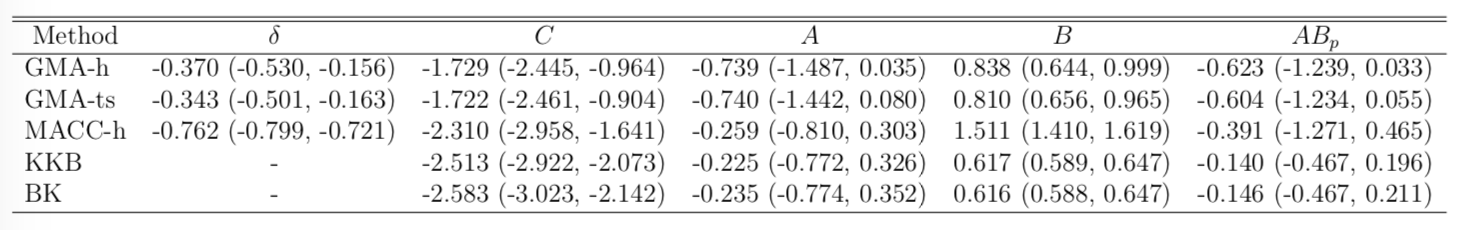• STOP deactivates M1 directly ($C$) and indirectly ($AB$)
• preSMA mediates a good portion of the total effect
• Help resolve the debates among neuroscientists
• Other methods under-estiamte the effects
• Novel feedback findings: M1 → preSMA after lag 1 and 2 (not shown)

## Discussion

• Mediation analysis for multiple time series
• Method: Granger causality + mediation
• Optimizing complex likelihood
• Theory: identifiability, consistency
• Result: low bias and improved accuracy
• Extension: functional mediation
• Paper in Biometrics 2019
• CRAN pkg: gma and references within

# Covariate Assisted Principal regression

## Co-AuthorsYi Zhao
Indiana Univ BiostatBingkai Wang
Johns Hopkins BiostatStewart Mostofsky
Johns Hopkins MedicineBrian Caffo
Johns Hopkins Biostat

## Motivating ExampleBrain network connections vary by covariates (e.g. age/sex)

Goal: model how covariates change network connections

$$\textrm{function}(\textbf{graph}) = \textbf{age}\times \beta_1 + \textbf{sex}\times \beta_2 + \cdots$$

## Resting-state fMRI Networks• fMRI measures brain activities over time
• Resting-state: "do nothing" during scanning

• Brain networks constructed using cov/cor matrices of time series## Mathematical Problem

• Given $n$ (semi-)positive matrix outcomes, $\Sigma_i\in \real^{p\times p}$
• Given $n$ corresponding vector covariates, $x_i \in \real^{q}$
• Find function $g(\Sigma_i) = x_i \beta$, $i=1,\dotsc, n$
• In essense, regress positive matrices on vectors

## Some Related Problems

• Heterogeneous regression or weighted LS:
• Usually for scalar variance $\sigma_i$, find $g(\sigma_i) = f(x_i)$
• Goal: to improve efficiency, not to interpret $x_i \beta$
• Covariance models Anderson, 73; Pourahmadi, 99; Hoff, Niu, 12; Fox, Dunson, 15; Zou, 17
• Model $\Sigma_i = g(x_i)$, sometimes $n=i=1$
• Goal: better models for $\Sigma_i$
• Multi-group PCA Flury, 84, 88; Boik 02; Hoff 09; Franks, Hoff, 16
• No regression model, cannot handle vector $x_i$
• Goal: find common/uncommon parts of multiple $\Sigma_i$
• Tensor-on-scalar regression Li, Zhang, 17; Sun, Li, 17
• No guarantees for positive matrix outcomes

## Massive Edgewise Regressions

• Intuitive method by mostly neuroscientists
• Try $g_{j,k}(\Sigma_i) = \Sigma_{i}[j,k] = x_i \beta$
• Repeat for all $(j,k) \in \{1,\dotsc, p\}^2$ pairs
• Essentially $O(p^2)$ regressions for each connection
• Limitations: multiple testing $O(p^2)$, failure to accout for dependencies between regressions

## $\mbox{PCA}(\Sigma_i) = x_i \beta$

• Essentially, we aim to turn unsupervised PCA to a supervised PCA
• Ours differs from existing PCA methods:
• Supervised PCA Bair et al, 06 models scalar-on-vector

# Model and Method

## Model

• Find principal direction (PD) $\gamma \in \real^p$, such that: $$\log({\gamma}^\top\Sigma_{i}{\gamma})=\beta_{0}+x_{i}^\top{\beta}_{1}, \quad i =1,\dotsc, n$$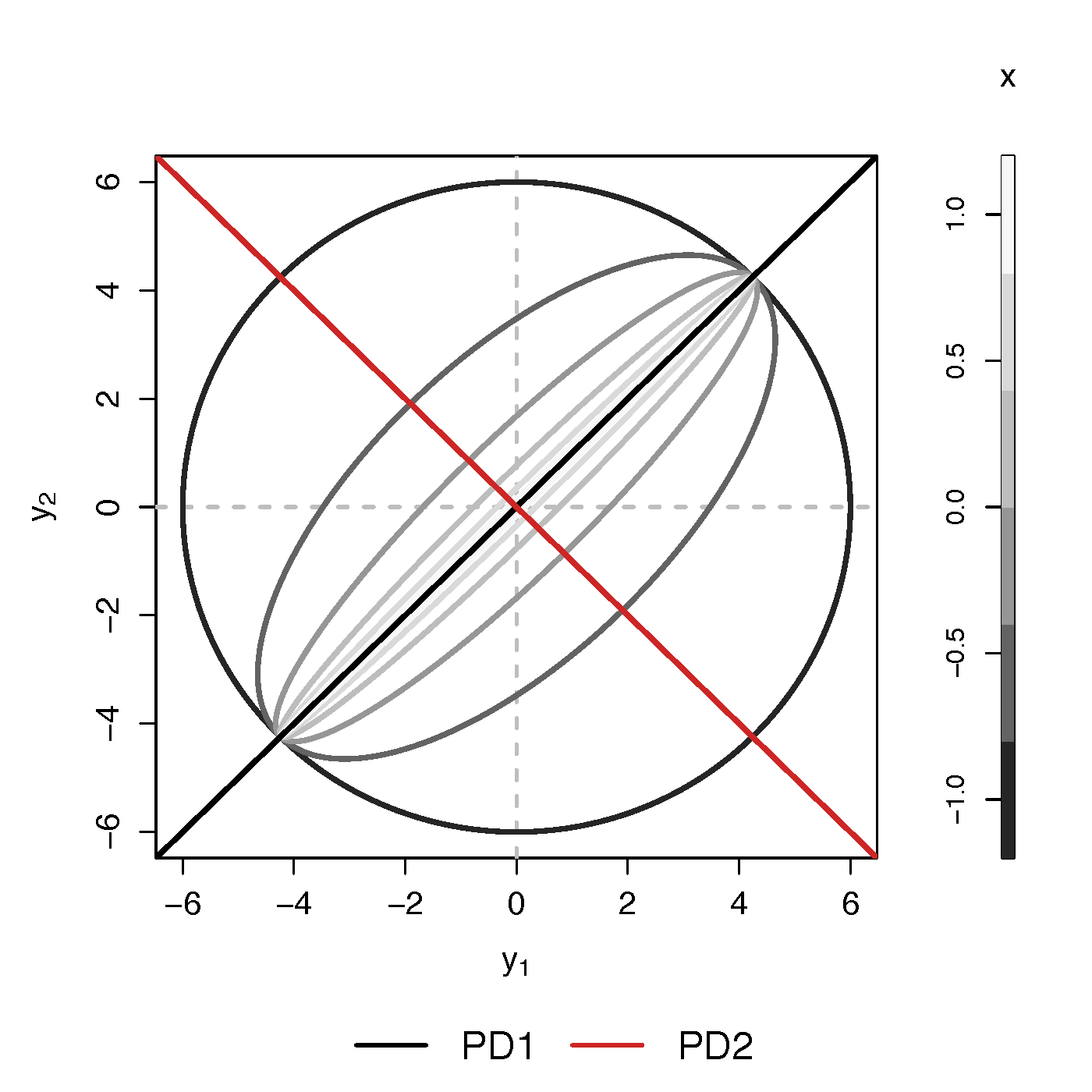Example (p=2): PD1 largest variation but not related to $x$

PCA selects PD1, Ours selects PD2

## Advantages

• Scalability: potentially for $p \sim 10^6$ or larger
• Interpretation: covariate assisted PCA
• Turn unsupervised PCA into supervised
• Sensitivity: target those covariate-related variations
• Covariate assisted SVD?
• Applicability: other big data problems besides fMRI

## Method

• MLE with constraints: $$\scriptsize \begin{eqnarray}\label{eq:obj_func} \underset{\boldsymbol{\beta},\boldsymbol{\gamma}}{\text{minimize}} && \ell(\boldsymbol{\beta},\boldsymbol{\gamma}) := \frac{1}{2}\sum_{i=1}^{n}(x_{i}^\top\boldsymbol{\beta}) \cdot T_{i} +\frac{1}{2}\sum_{i=1}^{n}\boldsymbol{\gamma}^\top \Sigma_{i}\boldsymbol{\gamma} \cdot \exp(-x_{i}^\top\boldsymbol{\beta}) , \nonumber \\ \text{such that} && \boldsymbol{\gamma}^\top H \boldsymbol{\gamma}=1 \end{eqnarray}$$
• Two obvious constriants:
• C1: $H = I$
• C2: $H = n^{-1} (\Sigma_1 + \cdots + \Sigma_n)$

## Choice of $H$

Proposition: When (C1) $H=\boldsymbol{\mathrm{I}}$ in the optimization problem, for any fixed $\boldsymbol{\beta}$, the solution of $\boldsymbol{\gamma}$ is the eigenvector corresponding to the minimum eigenvalue of matrix $$\sum_{i=1}^{n}\frac{\Sigma_{i}}{\exp(x_{i}^\top\boldsymbol{\beta})}$$

Will focus on the constraint (C2)

## Algoirthm

• Iteratively update $\beta$ and then $\gamma$
• Prove explicit updates
• Extension to multiple $\gamma$:
• After finding $\gamma^{(1)}$, we will update $\Sigma_i$ by removing its effect
• Search for the next PD $\gamma^{(k)}$, $k=2, \dotsc$
• Impose the orthogonal constraints such that $\gamma^{k}$ is orthogonal to all $\gamma^{(t)}$ for $t\lt k$

## Theory for $\beta$

Theorem: Assume $\sum_{i=1}^{n}x_{i}x_{i}^\top/n\rightarrow Q$ as $n\rightarrow\infty$. Let $T=\min_{i}T_{i}$, $M_{n}=\sum_{i=1}^{n}T_{i}$, under the true $\boldsymbol{\gamma}$, we have \begin{equation} \sqrt{M_{n}}\left(\hat{\boldsymbol{\beta}}-\boldsymbol{\beta}\right)\overset{\mathcal{D}}{\longrightarrow}\mathcal{N}\left(\boldsymbol{\mathrm{0}},2 Q^{-1}\right),\quad \text{as } n,T\rightarrow\infty, \end{equation} where $\hat{\boldsymbol{\beta}}$ is the maximum likelihood estimator when the true $\boldsymbol{\gamma}$ is known.

## Theory for $\gamma$

Theorem: Assume $\Sigma_{i}=\Gamma\Lambda_{i}\Gamma^\top$, where $\Gamma=(\boldsymbol{\gamma}_{1},\dots,\boldsymbol{\gamma}_{p})$ is an orthogonal matrix and $\Lambda_{i}=\mathrm{diag}\{\lambda_{i1},\dots,\lambda_{ip}\}$ with $\lambda_{ik}\neq\lambda_{il}$ ($k\neq l$), for at least one $i\in\{1,\dots,n\}$. There exists $k\in\{1,\dots,p\}$ such that for $\forall~i\in\{1,\dots,n\}$, $\boldsymbol{\gamma}_{k}^\top\Sigma_{i}\boldsymbol{\gamma}_{k}=\exp(x_{i}^\top\boldsymbol{\beta})$. Let $\hat{\boldsymbol{\gamma}}$ be the maximum likelihood estimator of $\boldsymbol{\gamma}_{k}$ in Flury, 84. Then assuming that the assumptions are satisfied, $\hat{ \boldsymbol{\beta}}$ from our algorithm is $\sqrt{M_{n}}$-consistent estimator of $\boldsymbol{\beta}$.

# Simulations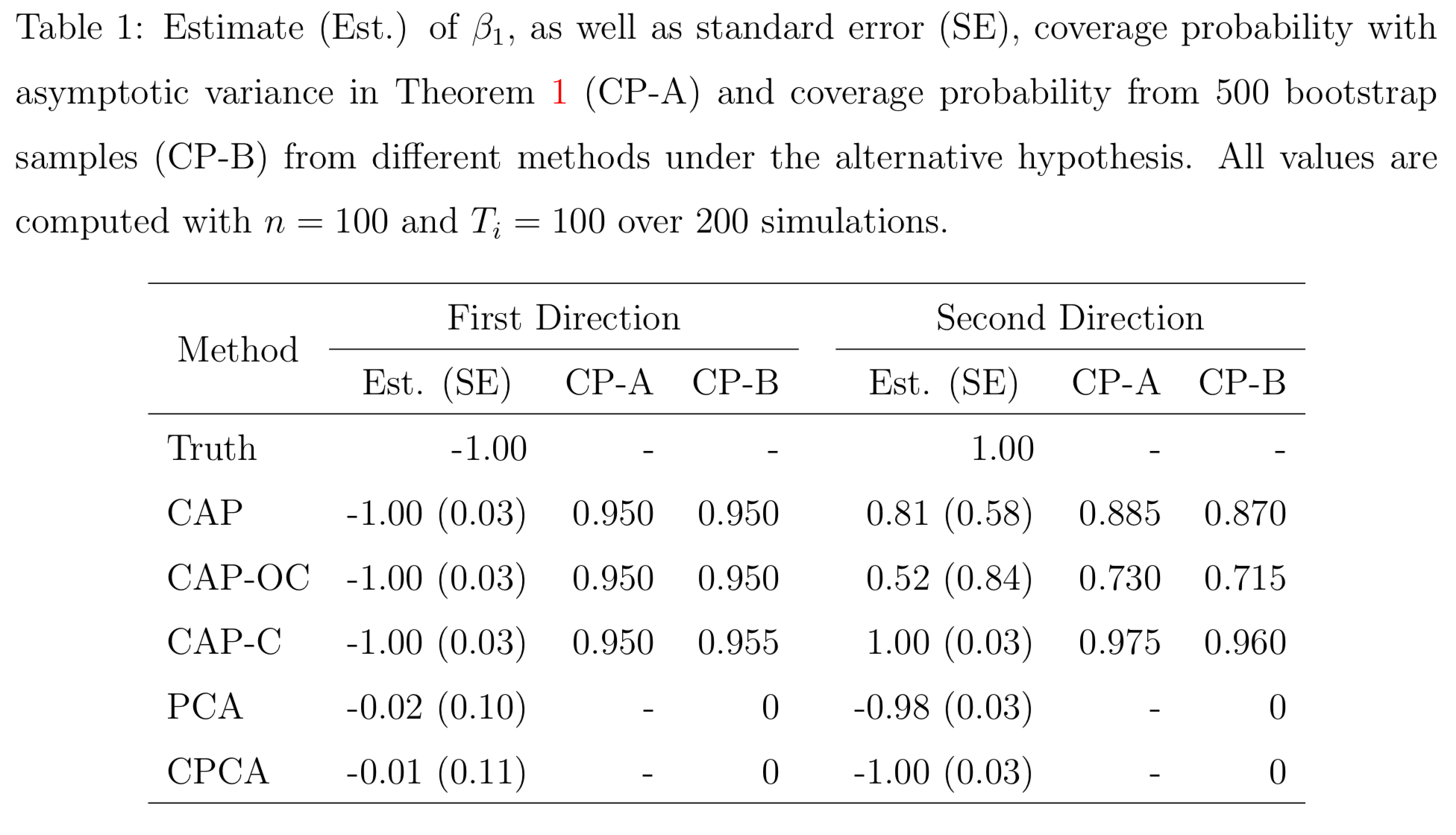PCA and common PCA do not find the first principal direction, because they don't model covariates

# Resting-state fMRI

## Regression Coefficients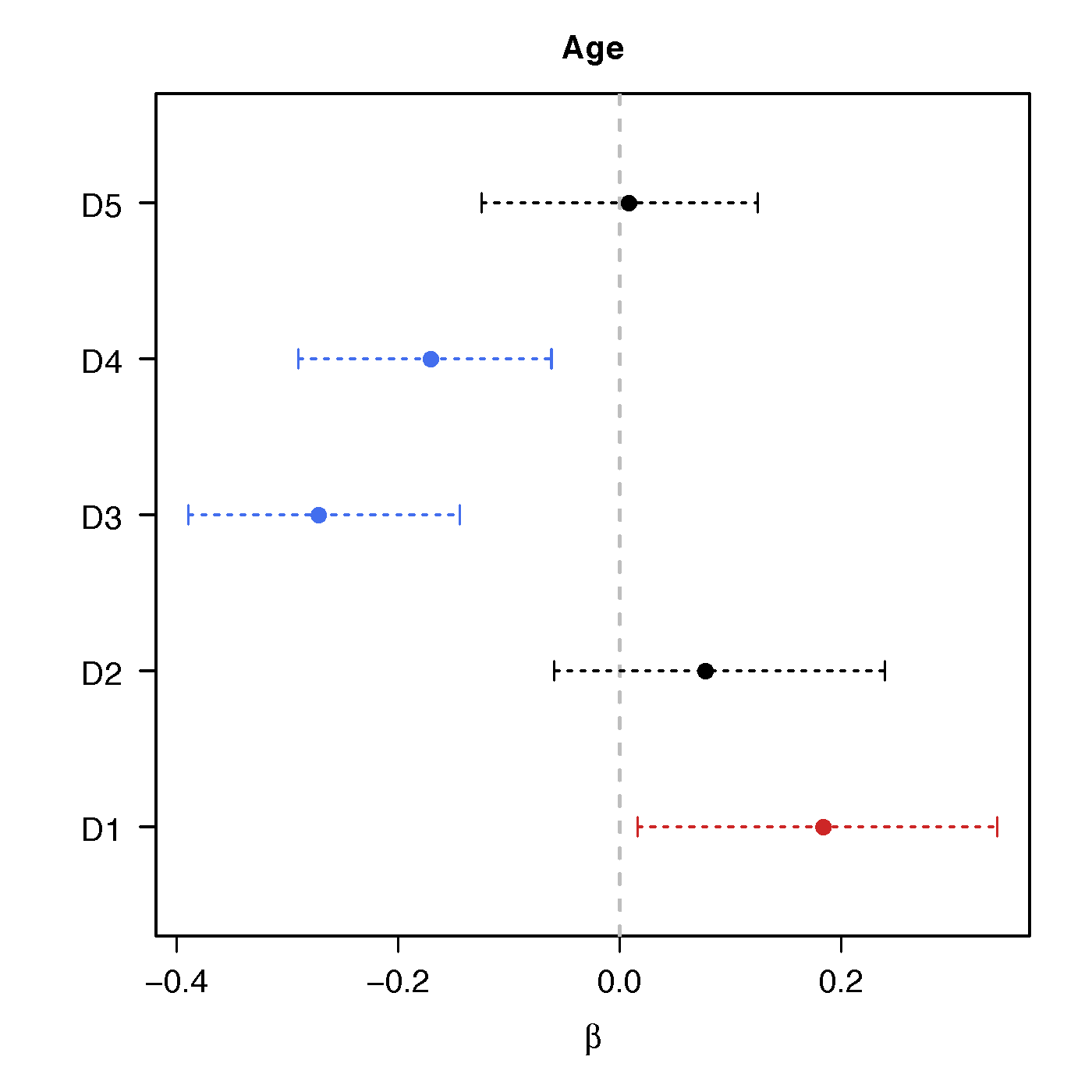AgeSex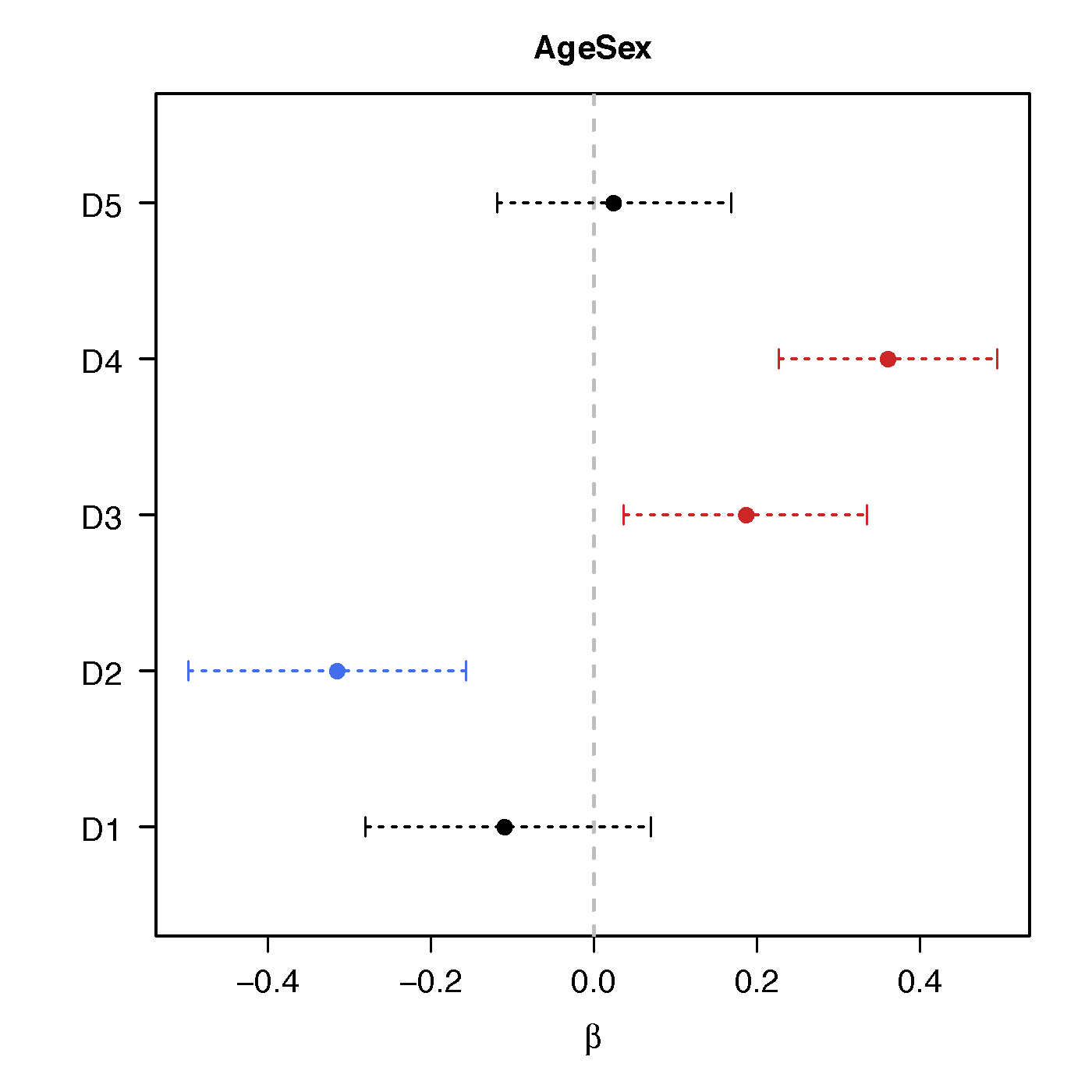Age*Sex

No statistical significant changes were found by massive edgewise regression

## Statistical Power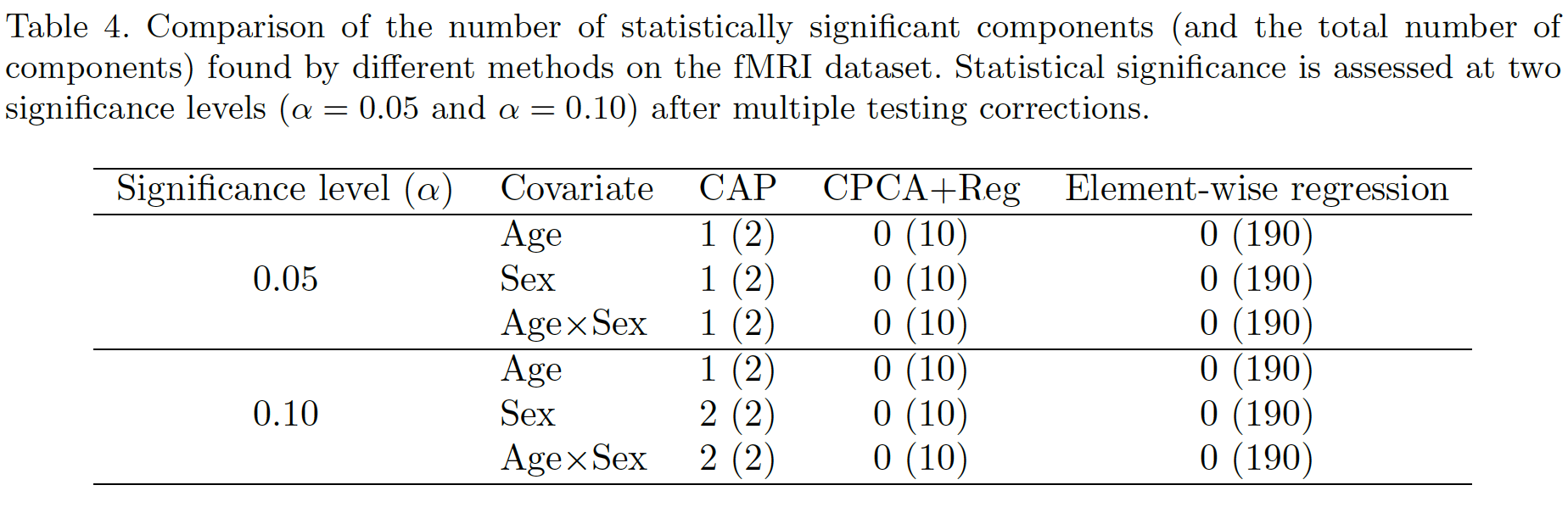## Brain Map of $\gamma$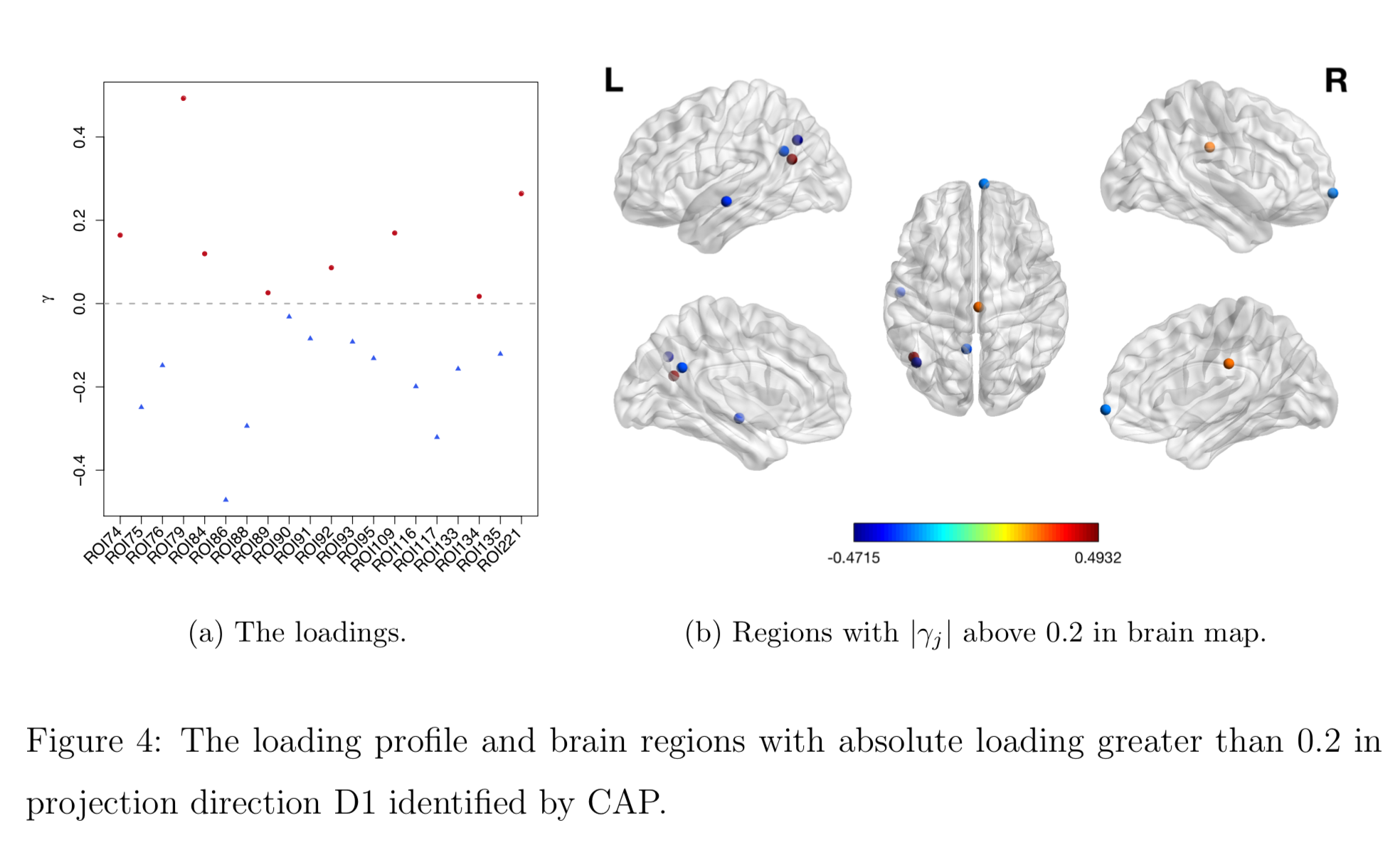## Discussion

• Regress matrices on vectors
• Method to identify covariate-related directions
• Theorectical justification
• Manuscript: DOI: 10.1101/425033 (Revision for Biostatistics)
• R pkg: cap# Thank you!

## Comments? Questions?

BigComplexData.com

or BrainDataScience.com